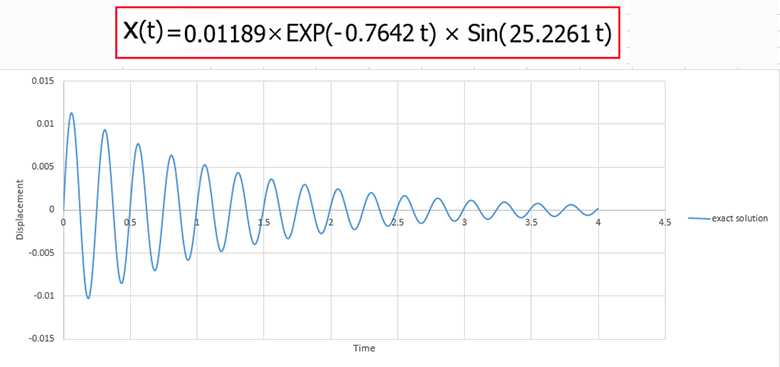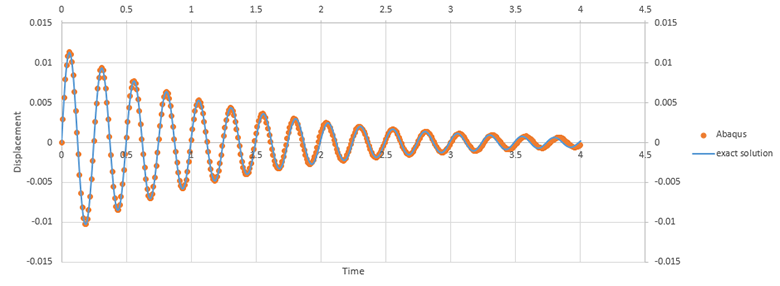پکیج آموزش پیشرفته آباکوس
پکیج جامع آباکوس ویژه رشته مکانیک
پکیج جامع آباکوس ژئوتکنیک و عمران

Free vibration of the single degree of freedom mass-spring-damper system using exact solution and abaqus

In this example we intend to simulate the free vibration of the single degree of freedom system in the Abaqus software. Actually we are going to obtain the equation of motion for the single degree of freedom mass-spring-damper system using the Abaqus software and exact solution and compare the outcomes having been obtained from the Abaqus software with those gained from the exact solution

As you know , if Zedta is smaller than 1 , the system will be called Underdamped . Indeed if Zedta is lower than 1 , it indicates that the system has an oscillating motion

We intend to solve the question when the Zedta is smaller than 1equation of motion from exact solutionequation of motion from AbaqusComparison between exact solution and Abaqus

As you know , if Zedta is bigger than 1 , the system will be called Overdamped . Indeed if Zedta is bigger than 1 , it indicates that the system will have no oscillating motion

We intend to solve the question when the Zedta is bigger than 1equation of motion from exact solutionequation of motion from AbaqusComparison between exact solution and Abaqus

Duration :37 minutes

The language of the video : English

Download link : full video , inp file , cae file , excel files

Payment Method : Paypal

To purchase this tutorial , please contact us

Email : saeedofmoeini@gmail.com